CBSE Sample Papers for Class 12 Computer Science Paper 4 are part of CBSE Sample Papers for Class 12 Computer Science. Here we have given CBSE Sample Papers for Class 12 Computer Science Paper 4.

## CBSE Sample Papers for Class 12 Computer Science Paper 4

 Board CBSE Class XII Subject Computer Science Sample Paper Set Paper 4 Category CBSE Sample Papers

Students who are going to appear for CBSE Class 12 Examinations are advised to practice the CBSE sample papers given here which is designed as per the latest Syllabus and marking scheme, as prescribed by the CBSE, is given here. Paper 4 of Solved CBSE Sample Paper for Class 12 Maths is given below with free PDF download Answers.

Time: 3 Hours
Maximum Marks: 70

General Instructions:

• All questions are compulsory within each Section.
• Programming Language in SECTION A : C++.

SECTION A

Question 1.
(a) Find out the correct identifiers out of the following, which can be used for naming variables, constants or functions in a C++ program.

File-Rec, 2abc, _DSP, D.9_7, My_2_3_90, if, getch, For, totalNum

(b) Meenu has started learning C++ and has typed the following program. When she compiled the following code written by her, she discovered that see needs to include some header files to successfully compile and execute it. Write the name of those header files, which are required to be included in the code.

void main()
{
char C, String[] = "Exercise Question1";
for(int i=0; String[i]!='\0'; i++)
if(isdigit(String[i])!= 0)
cout< else
cout<<String[i];
}

(c) Observe the following C++ code carefully and rewrite the same after removing all the syntax error(s) present in the code. Ensure that you 5 underline each correction in the code.
Note: Assume all the desired header files are already being included, which are required to run the code.
Correction should not change the logic of the program.

void main()
{
Future=55, Past=45;
Assign(Future; Past);
Assign(Past);
}
void Assign(int Default1, Default2=50)
{
Default1 = Default1+Default2;
cout<>Default2;
}

(d) Give the output of the following program code:

void displaylchar *S)
{
for(int a=0; S[a]>0; a++)
{
for(int Y=0; Y<=a; Y++)
cout<<S[Y];
cout<<endl;
}
}
void main()
{
char *t = "DARK";
display(t);
}

(e) Find and write the output of the following program code:
Note: Assume all required header files are already being included in the program.

class String
{
char Text[];
int Count;
public:
void Change(char Text[],int &Count)
{
char *Ptr = Text;
int len = strlen(Text);
for(;Count<len-2; Count+=2, Ptr++)
{
*(Ptr+Count) = tolower(*(Ptr+Count));
}
}
};
void main()
{
clrscr();
String S;
int Position = 1;
char Message!!] = "POINTERS MAGIC";
S.Change(Message,Position);
cout<<Message<<"@"<<Position;
}

(f) Observe the following program and find out, which output(s) out of (i) to (iv) will be expected from the program? Also, find out the minimum and the maximum value that can be assigned to the variable Points used in the code. Note: Assume all required header files are already being included in the program. random(n) function generates an integer between 0 to n-1

const int LIMIT = 4;
void main()
{
randomize();
int Points;
Points = 100 + random(LIMIT);
for(int P=Points; P>=100; P--)
cout<<P<<"#";
}

(i) 100#101#102#103#104
(ii) 103#102#101#100#
(iii) 100#101#102#103
(iv) 104#101#102#103#

Question 2.
(a) Define the term of Inheritance. Give an example in C++ to illustrate it.
(b) Answer the following questions (i) and (ii) after going through the following class:

class Science
{
char Topic;
int Weightage;
public:
Science() // Function1
{
strcpy(Topic,"Optics");
Weightage = 30;
cout<<"Topic Activated":
}
~Science() // Function2
{
cout<<"Topic Deactivated":
}
};

(i) Name the specific features of class shown by Function1 and Function2 in the above example.
(ii) How would Function1 and Function2 get executed?
(c) Define a class Cricket in C+ + with the following description:
Private members

• Target_score of type integer
• Overs bowled of type integer
• Extra time of type integer
• A penalty of type integer
• Cal_Penalty()
a member function to calculate penalty as follows:
if Extra_time<=10, Penalty=1 if Extra_time>10 but<=20, Fenalty=2 otherwise, Penalty=5

Public members

• A function Enterdata() to allow user to enter values for Target_score, Overs_bowled, Extra_time. Also, this function should call Cal_Penalty() to calculate penalty.
• A function Dispdata() to allow user to view the contents of all data members.

(d) Consider the following code and answer the questions given below:

class CHead
{
double Turnover:
protected:
int noofcomp;
public:
void INPUT(int);
void OUTPUT();
};
{
int noofemp;
protected:
float funds:
public:
Director();
void INDATA();
void OUTDATA();
};
class Manager: public Director
{
float Expense;
public;
Manager();
void DISPLAY(void);
};

(i) Which type of Inheritance out of the following is illustrated in the above example?
I. Single Level Inheritance
II. Multi Level Inheritance
III. Multiple Inheritance
(ii) How many bytes will an object belonging to class Manager required?
(iii) Name the member function(s), which are directly accessible from the object of class Manager.
(iv) Is the member function OUTPUT() accessible by the object of the class Director?

Question 3.
(a) Write a function check () to check, if the passed array of integers is sorted or not. The function should return 1 if arranged in ascending order, -1 if arranged in descending order, 0 if it is not sorted.
(b) An array S is stored in the memory along the row with each of the elements occupying 4 bytes. Find out the base address, if an element S  is stored at the memory location 7200.
(c) Each node of a STACK contains the following information, in addition pointer field
(i) Pincode of city
(ii) Name of city
Give the structure of node for the linked STACK in question. TOP is a pointer that points to the topmost node of the STACK.
To remove a node from the STACK, which is dynamically allocated STACK of items considering the following code is already written as a part of the program.

struct node
{
int Pincode;
char city;
};
class STACK
{
node *T0P;
public:
STACK()
{
TOP=NULL;
}
void PUSH();
void POP();
STACK();
};

(d) Evaluate the following postfix expression using a stack. Show the contents of stack after execution of each operation.
20, 8, 4, /, 2, 3, + , *, –
(e) Write a function in C++ to find sum of rows from a two dimensional array.
e.g. if two dimensional array contains
8 7 2
5 1 0
4 8 3
Output
Sum of row 1 is : 17
Sum of row 2 is : 6
Sum of row 3 is : 15

Question 4.
(a) Find the output of the following C++ code considering that the binary file “School.dat” exists on the hard disk with a data of 100 members.

#include
#include
#include
class School
{
int Scode;
char Name;
public:
void Input();
void Output();
};
void main()
{
ifstream f:
f.open("School.dat", ios::binary | ios :: in);
School S;
int POS=f.tellg()/sizeof (S);
cout<<"\nPresent Record:"<<POS<<endl;
f.close();
getch();
}

(b) Write a function in C++ to count the number of alphabets present in a text file “STORY. TXT”. e.g. If the file contains Computer science is the scientific and practical approach to computation and its application. It is the systematic study of the feasibility, structure, expression and mechanization of the methodical procedures. The number of alphabets: 179
(c) Assuming the class Computer as follows:

class Computer
{ char chiptype;
int Speed;
public:
void getdetails()
{
gets(chiptype);
cin>>Speed;
}
void showdetails()
{
cout<<"Chip"<<chiptype<<"Speed="<<Speed;
}
};

Write a function readfile() to read all the records present in an already existing binary file “COMP.DAT” and display them on the screen, also count the number of records present in the file.

SECTION B

Question 5.
(a) Observe the following tables Employee and Manager carefully and write the name of the RDBMS operation which will be used to produce the output as shown in “Output”. Also, find the degree and cardinality of the “Output”.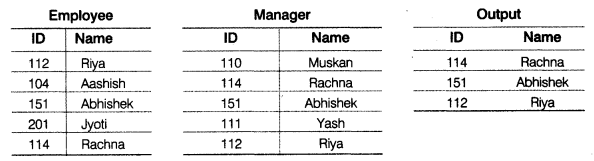(b) Write SQL queries for (i) to (iv) and find outputs for queries (v) to (viii) on the basis of the tables MOBILE and VENDOR(i) To display the names of all Samsung mobile users.
(ii) To display customer name and amount of all those mobiles whose amount is more than 800.
(iii) To display the number of users for each connection, i.e. the expected output should be
101 2
102 3
103 2
104 2
105 1
(iv) To display Cname, Model and Validity in decreasing order of Validity.

(v) SELECT COUNT(DISTINCT Model) FROM MOBILE;
(vi) SELECT MAX(Activation), MIN(Activation) FROM MOBILE;
(vii) SELECT A.Connection, B.Cname, B.Amount FROM VENDOR A,MOBILE B
WHERE A.CCode=B.CCode AND Cname LIKE 'r%'
(viii) SELECT Benefits, Cname, Activation FROM VENDOR, MOBILE
WHERE VENDOR.CCode=MOBILE.Ccode
AND Activation BETWEEN '2004-05-14' AND '2004-06-10';

Question 6.
(a) Verify the following algebraically:
$$\left( \bar { A } +\bar { B } \right) \cdot \left( A+B \right) =\bar { A } \cdot B+A\cdot \bar { B }$$
(b) Write the Boolean expression for the result of the logic circuit as shown below: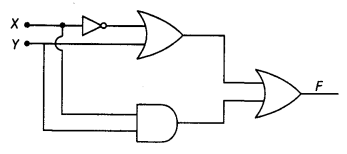(c) Write the canonical SOP form of a Boolean function F, which is represented in a truth table as follows: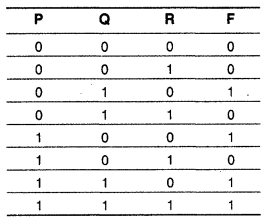(d) Reduce the following Boolean expression using K-map
F(u, v, w, z) = Π (0, 1, 2, 4, 5, 6, 8, 10)

Question 7.
(a) How does CDMA technique works?
(b) Anuradha is a Web developer. She has designed a login form to input the login id and password of the user. She has to write a script to check whether the login id and the corresponding password as entered by the user are correct or not. What kind of script from the following will be most suitable for doing the same?
(i) JSP
(ii) Client-Side Script
(iii) VB Script
(c) How is wi-fi different from wi-max?
(d) What do you mean by IP address? How is it useful in computer security?
(e) Computer Ltd has set-up its new center at Meerut for its office and Web-based activities. It has 4 blocks of buildings as shown in the diagram below: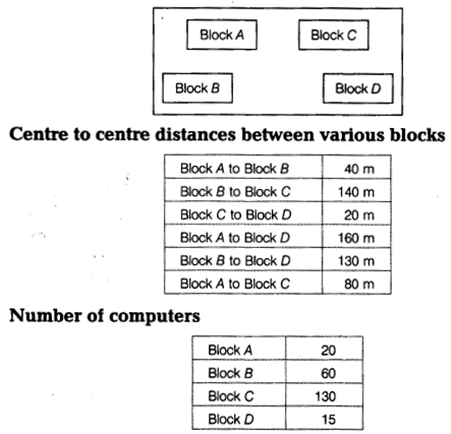(i) Suggest a cable layout of connections between the blocks.
(ii) Suggest the most suitable place (i.e. block) to house the server. Give reason also.
(iii) Suggest the placement of repeater device with justification.
(iv) The organization is planning to link its front office situated in the city in a hilly region where the cable connection is not feasible, suggest an economical way to connect it with reasonably high speed.
(f) list any two disadvantages of the star topology.
(g) How is a hacker different from a cracker?

(a) Correct identifiers are: _DSP, My_2_3_90, For, totalNum

(b) → cout()
→ isdigit()
(c) The correct code is:
void Assian(int Default1. int Default2=50):
void main()
{
int Future=55, Past=45;
Assiqn(Future, Past):
Assign(Past);
}
void Assign(int Default1, int Default2)
{
Default1=Default1+Default2;
cout<<Default1<<Default2:
}

(d) Output
D
DA
DAR
DARK
(e) Output
PoINtERs MaGIc@13
(f) Possible output is
(ii) 103#102#101#100#
The minimum value of variable Points = 100
The maximum value of variable Points = 103

(a) Inheritance is the capability to inherit the properties from another class. The class that derives its properties is called derived class or child class and the class from which the properties are derived is called the base class or parent class. The child class can access all properties and behaviors, the child class along with the inherited properties can possess its own properties. In other words, inheritance is a way of code reusability.
Inheritance can be done by using the following syntax:

class :
{
..........
.........
};

The following program illustrates the use of inheritance:
e.g.

#include
#include
class Parent
{
public;
int num;
void fun()
{
num=10;
cout<<num;
}
};
class Child:public Parent
{
void fun1()
{
num = 20;
cout<<num;
}
};
void main()
{
Child obj;
//child class object calling base class function
obj.fun();
}

(b) (i) Specific features of class shown by Function 1 is the constructor and by Function2 is destructor.
(ii) Whenever an object of class Science is created, Function1 will be called automatically, similarly, Function2 will be invoked automatically whenever Science class object goes out of scope.

(c) class Cricket
{
private:
int Target_score;
int Overs_bowled;
int Extra_time;
int Penalty;
void Cal_Penalty()
{
if(Extra_time <= 10)
Penalty = 1;
else if(Extra_time <= 20)
Penalty = 2;
else
Penalty = 5;
}
public:
void Enterdata()
{
cout<<"Enter Target Score\n"; cin>>Target_score;
cout<<"\nEnter overs bowled": cin>>Overs_bowled;
cout<<"\nEnter Extra Time"; cin>>Extra_time;
Cal_Penalty();
}
void Dispdata()
{
cout<<"Target Score:"<<Target_score;
cout<<"\nOvers bowled:"<<0vers_bowled;
cout<<"\nExtra Time:"<<Extra_time;
cout<<"Penalty:"<<Penalty;
}
};

(d) (i) II. Multi Level Inheritance
(ii) Object belonging to class Manager will require 20 bytes.
(iii) The member functions which are directly accessible from the object of class Manager are as follows: DISPLAY(), INDATA(), OUTDATA(), INPUT(), OUTPUT()
(iv) Yes, the member function OUTPUT() is directly accessible by the object of class Director.

(a) int check(int a[], int n) { int f=0, i = 0; if(a[i]>a[i+1])
{
f=-1;
for(i = 1; i<n-1; i++)
{
if(a[i ]<a[i +1])
{
f=0;
break;
}
}
}
el se
{
f = 1;
for(int i=1; i< n-1; i++) { if(a[i]>a[i+1])
{
f =0;
break;
}
}
}
return f;
}

(b) M= 30, N= 20 and W = 4 bytes
S = Base + [N*(I-0)+(J-0)] *W
7200 = Base + [20*(15 – 0) + (10 – 0)]* 4
7200 = Base + [300+10]* 4
7200 = Base + * 4
7200 = Base + 1240
Base = 7200 – 1240
Base = 5960

(c) void POP(node *T0P; int Pin, char Mcity[])
{
node *temp;
char Mcity;
if(TOP==NULL)
cout<<"\nError!!Stack is Empty!!"; else { temp = TOP; TOP = TOP—> link;
Pin = temp->Pincode;
strcpy(Mcity, temp->City);
delete temp;
}
return(TOP);

(d) The postfix expression 20, 8, 4, /, 2, 3, +, *, –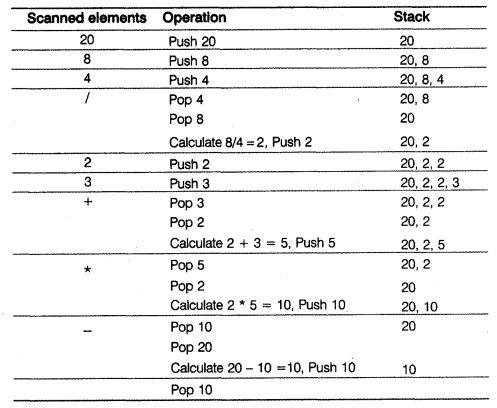Result = 10

(e) void sumrow(int A[], int n, int m)
{
for(int p=0; p<n; p++)
{
int sum = 0;
for(int q=0; q<m; q++)
sum+= A[p][q];
cout<<"Sum of row "<<p+1<<" is: "<<sum;
}
}

(a) Present Record : 2

(b) void Count()
{
ifstream fin("STORY.TXT", ios::in);
int n=0;
char ch = fin.get();
while(!fin.eof())
{
if(isalpha(ch))
n++;
ch = fin.get();
}
cout<<"Number of alphabets:"<<n<<endl;
}
{
int i=0;
ifstream file;
fi1e.open("COMP.DAT", ios::binary | ios::in);
Computer c;
{
c.showdetais();
i = i+1;
}
cout<<"Number of records present"<<i;
file.close();
}

(a) RDBMS operation is Intersection Degree = 2 Cardinality = 3

(b) (i) SELECT Cname FROM MOBILE WHERE Model = 'Samsung';
(ii) SELECT Cname. Amount FROM MOBILE WHERE Amount > 800;
(iii) SELECT CCode, COUNT(Cname) FROM MOBILE GROUP BY CCode;
(iv) SELECT Cname, Model. Validity FROM MOBILE ORDER BY Validity DESC;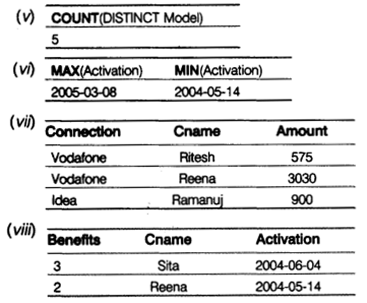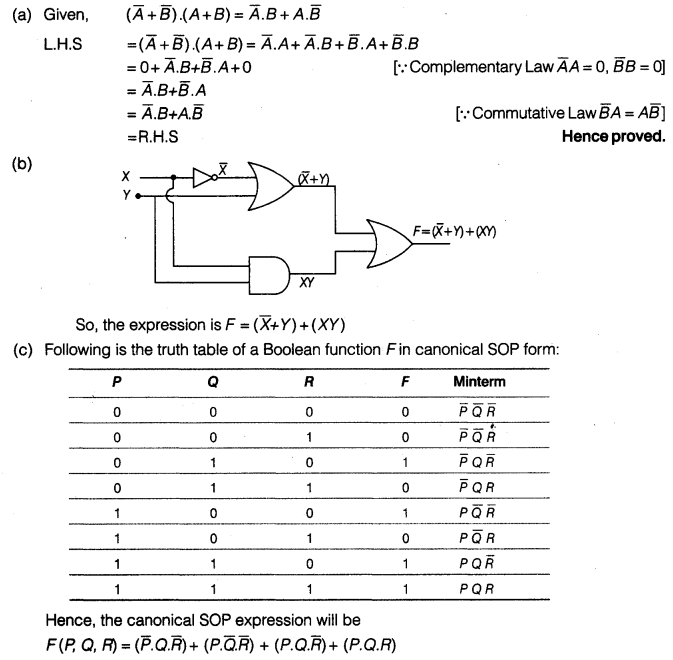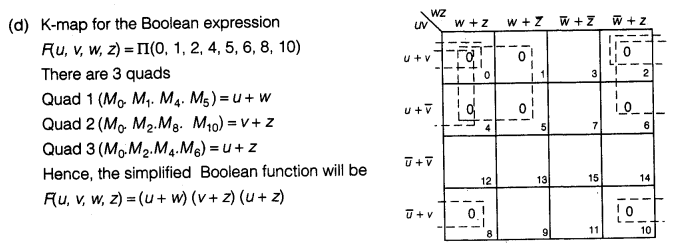(a) In the CDMA technique, the data is sent in small pieces over a number of discrete frequencies available for use at any time in the specified range.
(b) (i) JSP, as server-side scripting will be used.
(c) Differences between wi-fi and wi-max are as follows:

 wi-fi wi-max It is a short-range technology mostly used in, in-house applications. It is a long-range technology to deliver wireless broadband to the far end. It was CSMA/CA protocol which could be connection based or connectionless. It uses connection-oriented MAC protocol.

(d) An Internet Protocol (IP) address is a numerical identification and logical address that is assigned to devices connected in a computer network.
In a network, every machine can be identified by a unique IP address associated with it and thus, help in providing network security to every system connected in a network.
(e) (i) Cable layout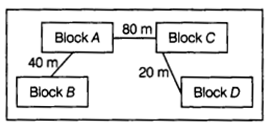(ii) The most suitable place to house the server is Block C as this block contains the maximum number of computers.
(iii) Since the distance between Block A and Block C is larger, so a repeater would ideally be placed in between this path.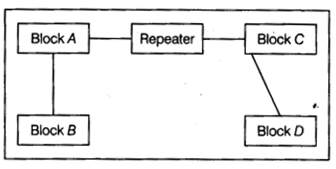(iv) The most economical way to connect it with a reasonably high speed would be to use radio waves transmission as it is easy to install as compared to other unguided media, can travel long distances and penetrate walls.
(f) Two disadvantages of star topology are as follows:
(i) It is difficult to expand.
(ii) If the central node of the network fails, the entire network is rendered inoperable.
(g) The crackers are malicious programmers who break into secure systems whereas hackers are more interested in gaining knowledge about computer systems and possibly using this knowledge for playful pranks.

We hope the CBSE Sample Papers for Class 12 Computer Science Paper 4 help you. If you have any query regarding CBSE Sample Papers for Class 12 Computer Science Paper 4, drop a comment below and we will get back to you at the earliest.